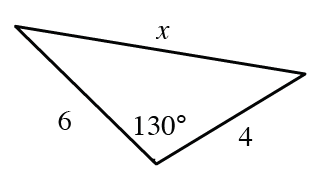### Home > INT3 > Chapter 10 > Lesson 10.2.2 > Problem10-134

10-134.

Consider the triangle at the right.

1. Solve for $x$ in the triangle.

You are working with $3$ sides and one angle. Use the Law of Cosines to find the value of $x$.

$x^{2} = \left(6\right)^{2} + \left(4\right)^{2} − 2\left(6\right)\left(4\right)\text{cos}\left(130°\right)$

$x = 9.10$

2. What is the area of the triangle?